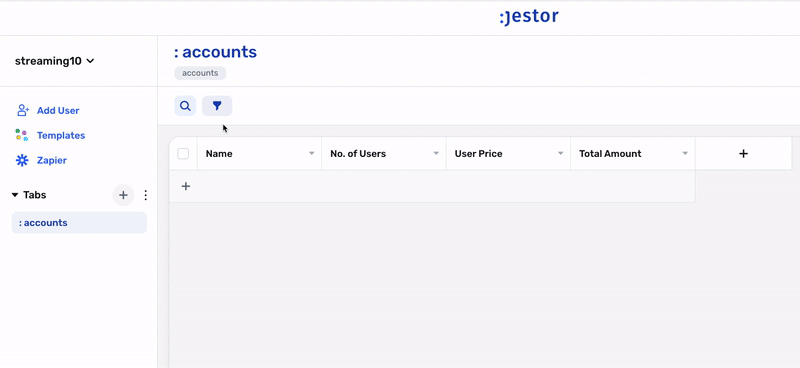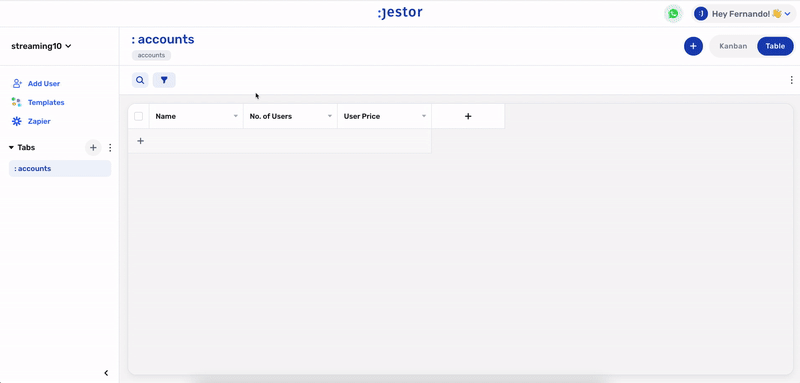# Basic Formulas

Automatically add, subtract, multiply or divide values

# What is the Basic Formulas field?

It's a field dedicated to the standard four operations: Addition, Subtraction, Division and Multiplication. This field automatically calculates a result based on two numeric fields, or a numeric field and a manually set value.# How to create a basic formula field

To create a Formula field:

• On table mode, click on the last table header with a "+" and that will open the create field page
• Just look for the "Basic Formulas" option and click on it
• Choose the name of your field and if it's a required field

### 📘

You can delete or edit by clicking on the table header name and choosing one of the options# When should I use it?

When you wish to create a field that automatically calculates a result based on the record's other fields, such as calculating an account's subscription value by multiplying user price by number of users.

What’s Next